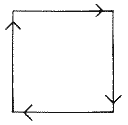`COMPUTE! ISSUE 57 / FEBRUARY 1985 / PAGE 10`

Apple Shape Tables
I have an Apple II+ and have been trying to figure out shape tables. How do the data numbers affect a shape? How do the numbers in the DRAW and XDRAW commands make a shape?
Tony Steele

Shape tables can appear very confusing, but they are extremely useful, though in some cases it may be easier to draw complicated figures with HPLOT.

Basically, a shape table contains plotting vectors to draw a figure. Each vector describes the movement necessary to draw the object.

Let's try constructing a shape table to draw a square to see how it all gets done. The first step is to draw the shape on a piece of paper.Now you must convert the figure to coded plotting vectors. Vector codes are numbers between 0 and 7 which correspond to a direction of movement, and each byte of a shape definition can hold as many as three vectors. The task now is to reduce the shape to a series of vectors, then place these vectors into memory, where they can be used to draw shapes.
Pick a starting point on the figure you want to code. For our square, we'll start at the bottom-left corner. Make a list of the directions required to draw the shape. Be sure you include all movements necessary, even those not actually drawn on the screen.
Starting at the bottom-left corner, we need these vectors to draw our square:

 Vector Plot up yes right yes down yes left yes

Now use this table to write the proper binary code next to each vector:

 Action Binary Code Decimal Code move up without plotting 000 0 move right without plotting 001 1 move down without plotting 010 2 move left without plotting 011 3 move up with plotting 100 4 move right with plotting 101 5 move down with plotting 110 6 move left with plotting 111 7

You should now have a table that looks like this:

 Vector Plot Code up yes 100 right yes 101 down yes 110 left yes 111

The Apple stores information in memory in bytes of eight-bit binary numbers. Usually two, and sometimes three, plotting vectors can be stored in a specific memory address. A memory byte can be thought of as:

 7 6 5 4 3 2 1 0 A B C

There are a few rules to follow when packing binary codes into memory:
1. All bytes are read from right to left.
2. When all remaining sections of a byte contain zeros, the rest of the byte will be ignored.
3. Only a move instruction may be placed in section A of a byte.
Now refer to your table and place the binary code for the first vector into section C of the first byte, and place the binary code of the second vector into section B of the byte.
In section A of each byte, a zero always means no movement and no plotting, and a zero value will be ignored by BASIC. The only legal vectors in section A are right, left, and down without plotting. No other plotting vectors are allowed in section A.
The byte should now look like this:

 7 6 5 4 3 2 1 0 A 0 0 B  1 0 1 C  1 0 0

Notice that the vector for move right with plotting was not placed into section A. Start filling in the next byte with the remaining values.

 7 6 5 4 3 2 1 0 A 0 0 0 0 0 0 B  1 0 1  1 1 1  0 0 0 C  1 0 0  1 1 0  0 0 0

This is the table for drawing the square. After each byte has been filled in, set the last byte to zero. This signals BASIC that the end of the shape table has been reached.
Divide the byte into two four-bit portions (nybbles) and convert the nybbles to hexadecimal numbers:

 Binary Hex 0 0 1 0    1 1 0 0 0 0 1 1    1 1 1 0 0 0 0 0    0 0 0 0 2 C 3 E 0 0

The only information needed to complete the shape table is the Shape Table Directory, which contains the number of shape definitions in the table and which points to a starting location for each shape.
The first byte of the shape table contains the number of shape definitions. The second byte is unused, and starting with the third byte, a table of indices to the starting addresses of each shape definition is stored. This value is the offset that must be added to the starting address of the table to obtain the starting address of a specific shape.
Using the example of our square, we'll store the shape table starting at \$1F00. The completed shape table looks like this:

1 F 0 0    0 1 \
1 F 0 1    0 0  > Directory
1 F 0 2    0 4 /
1 F 0 3    0 0\
1 F 0 4    2 C \
1 F 0 5    3 E  > Shape Definition
1 F 0 6    0 0 /

This shape table may be stored in memory using the monitor, or by POKEing the values from a table of DATA. The starting address of the shape table must be stored in memory location \$E8. Again, you can place it there with the monitor, or by entering the following in immediate mode:

POKE 232,0
POKE 233,31

That completes the shape table, and you're ready to save the table and DRAW, XDRAW ROT, or SCALE to your heart's content.